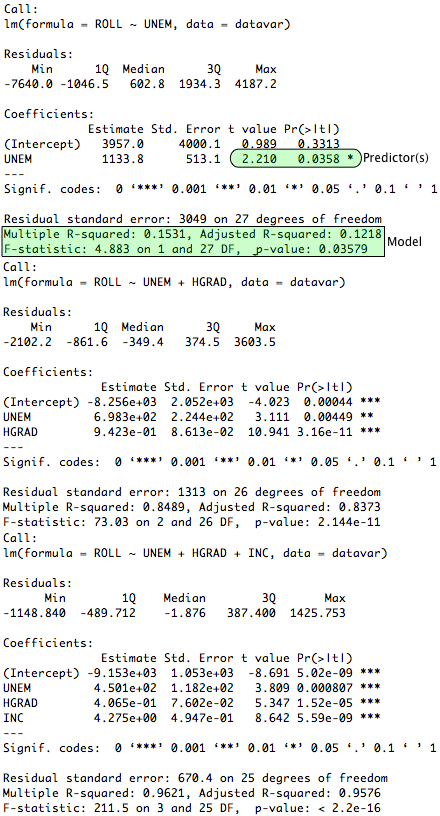[This article was first published on R Tutorial Series, and kindly contributed to R-bloggers]. (You can report issue about the content on this page here)
Want to share your content on R-bloggers? click here if you have a blog, or here if you don't.

Regression models can become increasingly complex as more variables are included in an analysis. Furthermore, they can become exceedingly convoluted when things such as polynomials and interactions are explored. Thankfully, once the potential independent variables have been narrowed down through theoretical and practical considerations, a procedure exists to help us identify which predictors make a significant statistical contribution to our model. Hierarchical linear regression (HLR) can be used to compare successive regression models and to determine the significance that each one has above and beyond the others. This tutorial will explore how the basic HLR process can be conducted in R.

### Tutorial Files

Before we begin, you may want to download the sample data (.csv) used in this tutorial (UPDATE: the data is no longer online. Try this link, it seems like the data, but will require more work to get it into csv format). Be sure to right-click and save the file to your R working directory. This dataset contains information used to estimate undergraduate enrollment at the University of New Mexico (Office of Institutional Research, 1990). Note that all code samples in this tutorial assume that this data has already been read into an R variable and has been attached.

### Pre-Analysis Steps

Before comparing regression models, we must have models to compare. In the segment on multiple linear regression, we created three successive models to estimate the fall undergraduate enrollment at the University of New Mexico. The complete code used to derive these models is provided in that tutorial. This article assumes that you are familiar with these models and how they were created. Therefore, a shorthand method for generating the models is displayed below.
```> #create three linear models using lm(FORMULA, DATAVAR)

> #one predictor model

> onePredictorModel <- lm(ROLL ~ UNEM, datavar)

> #two predictor model

> twoPredictorModel <- lm(ROLL ~ UNEM + HGRAD, datavar)

> #three predictor model

> threePredictorModel <- lm(ROLL ~ UNEM + HGRAD + INC, datavar)```

### Comparing Individual Models

The summary(OBJECT) function can be used to ascertain the overall variance explained (R-squared) and statistical significance (F-test) of each individual model, as well as the significance of each predictor to each model (t-test). The following code demonstrates how to generate summaries for each model.
```> #get summary data for each model using summary(OBJECT)
> summary(onePredictorModel)
> summary(twoPredictorModel)
> summary(threePredictorModel)```
The results of the previous functions are displayed below.From the summary functions, we can infer that all of the models are statistically significant. Moreover, each one explains more of the overall variance than the previous model. We can also assess the significance of the individual predictors to each equation. Note that, if preferred, similar comparisons could be made by using the anova() function on each model.

### Comparing Successive Models

The anova(MODEL1, MODEL2,… MODELi) function can be used to compare the significance of each successive model. The code sample below demonstrates how to use ANOVA to accomplish this task.
```> #compare successive models using anova(MODEL1, MODEL2, MODELi)

> anova(onePredictorModel, twoPredictorModel, threePredictorModel)```
The table resulting from the preceding function is pictured below.Here, we can see that each successive model is significant above and beyond the previous one. This suggests that each predictor added along the way is making an important contribution to the overall model.

### More HLR

Undoubtedly, HLR is a complex topic that has only been addressed at the most basic level in this tutorial. Further guides in the series will cover related subjects, such as interactions and polynomial regression. However, individuals whose work requires a deeper inspection into the procedures of HLR are encouraged to seek additional resources (and to consider writing a guest tutorial for this series).

### Complete Hierarchical Linear Regression Example

To see a complete example of how HLR can be conducted in R, please download the HLR example (.txt) file.

### References

Office of Institutional Research (1990). Enrollment Forecast [Data File]. Retrieved November 22, 2009 from http://lib.stat.cmu.edu/DASL/Datafiles/enrolldat.html (this URL is no longer active. You can try for a live version here)